# Hidden Markov Models: The Baum-Welch Algorithm

Suppose you are at a table at a casino and notice that things don’t look quite right. Either the casino is extremely lucky, or things should have averaged out more than they have. You view this as a pattern recognition problem and would like to understand the number of ‘loaded’ dice that the casino is using and how these dice are loaded. To accomplish this you set up a number of Hidden Markov Models, where the loaded die are the latent (hidden) variables, and would like to determine which of these, if any is more likely to be using.

First lets go over a few things.

We will call each roll of the dice an observation. The observations will be stored in variables o1, o2, …, oT, where T is the number of total observations.

To generate a hidden Markov Model (HMM)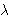we need to determine 5 parameters:

• The N states of the model, defined by S = {S1, …, SN}
• The M possible output symbols, defined by= {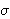1,2, …,M}
• The State transition probability distribution A = {aij}, where aij is the probability that the state at time t+1 is Sj, given that the state at time t is Si.
• The Observation symbol probability distribution B = {bj(k)} where bj(k) is the probability that the symbolk is emitted in state Sj.
• The initial state distribution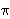= {i}, wherei is the probability that the model is in state Si at time t = 0.

The HMMs we’ve generated are based on two questions. For each question, you have provided 3 different answers which leads to 9 possible HMMs. Each of these models has its corresponding state transition and emission distributions.

• How often does the casino change dice?
• 0) Dealer Repeatedly Uses Same Dice
• 1) Dealer Uniformly Changes Die
• 2) Dealer Rarely Uses Same Dice
• Which sides on the loaded dice are more likely?
• 0) Larger Numbers Are More Likely
• 1) Numbers Are Randomly Likely
• 2) Smaller Numbers Are More Likely
How often does the casino change dice?
Which sides on
are more likely?
 (0, 0) (0, 1) (0, 2) (1, 0) (1, 1) (1, 2) (2, 0) (2, 1) (2, 2)

One of the interesting problems associated with Hidden Markov Models is called the Learning Problem, which asks the question “How can I improve a HMMso that it would be more likely to have generated the sequence O = o1, o2, …, oT?

The Baum-Welch algorithm answers this question using an Expectation-Maximization approach. It creates two auxiliary variables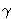t(i) and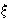t(i, j). The variablet(i) represents the probability of being in state i at time t, given the entire observation sequence. Likewiset(i, j) represents the joint probability of being in state i at time t and of being in state j at time t+1, given the entire observation sequence. They can be calculated byt(i) = (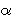t(i) *t(i) )j = 1 to N(t(j) *t(j))

andt(i, j) = (t(i) * ai, j *t+1(j) * bj(ot+1) )i’ = 1 to Nj’ = 1 to N(t(i’) * ai’, j’ *t+1(j’) * bj’(ot+1) )

As you can see, these are a direct result of calculations offrom the Forward algorithm andfrom the Backwards algorithm. Once we have calculated these variables, we can update the parameters of the model as follows: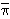i =1(i)i,j =t = 1 to T-1(t(i))t = t to T-1 (t(i, j))

// [b bar]_{j, k} = Sigma_{t = 1 to T, o_t = o_k} gamma_{t, j} / Sigma_{t = 1 to T} gamma_{t, j}, 1 <= j <= N, 1 <= k <= Mj(ok) =t = 1 to T-1, ot = okt(j)t = 1 to T-1t(j)

We can iterate this procedure a finite number of times or until it converges. This will generate a new model,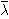= {N,,,,}.

There is more on this example at LEARNINGlover.com: Hidden Marokv Models: The Baum-Welch Algorithm.

Some further reading on Hidden Markov Models:

# Examples Page

Sometimes, the most effective way to understand a new concept is to actually see it in action. On My Examples Page, I have implemented a variety of scripts to help teach many different concepts.This is the page that is the easiest for me to update, so you will regularly see changes to this page along with an accompanying blog entry at My Blog Page.

This page is focused on teaching individual concepts and/or algorithms. In particular, with the HTML5 Canvas element, I’ve been able to visualize many of these concepts. Generally I try to provide a script that executes the given algorithm (or concept) and allows for users to view these concepts on random instances. When possible, I provide a button labeled “New Problem” (or something similar) which will allow the user to view a different instance of the algorithm.